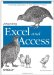# 5.5. Writing Excel Worksheet Functions from Access VBA

 < Day Day Up >

 You have already been exposed to the .FormulaR1C1 property of a cell in the created crosstab example earlier this chapter. When you use this property to write a function, the Excel worksheet stores it. When data changes in the Excel worksheet being referenced by the formula, the formula result also changes. Any functions that you can write from the Excel user interface can also be written from VBA. You can use either A1 or R1C1 reference style based on the formula property. For example, FormulaR1C1 and FormulaArray must be entered using R1C1 style, and the Formula property must use the A1 reference style. Set the workbook option to use labels in formulas from VBA and the FormulaLabel type for the range object. In addition to actually writing formulas that end up in Excel, you can also use the functions available in the WorksheetFunction property of the Excel application object to return a value and place that value in the Excel cell. For example, if you write: ` xlRng.FormulaR1C1 = "=Sum(R2C:R[-1]C)" ` you end up with a formula in the cell, but if you write: ` xlrng.Value = Excel.WorksheetFunction.Sum(xlws.Range(_ xlws.Cells(2, xlrng.Column), _ xlws.Cells(xlrng.Row - 1, xlrng.Column))) ` you end up with only the formula result in the cell. Depending on the purpose of the application, you might not want your users to see your formulas. In addition, you might not want the results of your formulas to change if a user changes the base numbers. Normally, I don't use the WorksheetFunctions unless I am calculating on the fly and won't want or need the formulas later. When I automate Excel from Access, though, I almost always use the actual formulas. If I am concerned about people changing the formulas, I can hide them, lock the rest of the worksheet, and protect the worksheet with a password. This way, I can go in later and make sure everything is calculating correctly without worrying about things changing that I didn't want to change.
 < Day Day Up >Integrating Excel and Access
ISBN: 0596009739
EAN: 2147483647
Year: 2005
Pages: 132

Similar book on Amazon